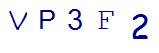# Try to Stump your Child with these Tough Fraction Word Problems for 6th GradeLast Updated on May 26, 2022 by Thinkster

Middle school brings challenging math concepts. For example, the fraction word problems 6th grade students often have to tackle will challenge even the most competent adult. If your child has entered the world of fraction word problems and is looking for a challenge, try to stump him with these problems.

## 1. Johnny has half a tablespoon of food coloring and a third a tablespoon of vanilla. How many more tablespoons of food coloring does he have than vanilla extract?

“How many more” is a clue word for subtraction. This problem requires your student to subtract 1/3 a tablespoon from 1/2 tablespoon. To do this problem, you must convert to a common denominator. This gives you 3/6 – 2/6. The answer, therefore, is 1/6 tablespoon. You can change the values in this sample word problem to practice subtraction in other ways.

## 2. Sarah has 2/3 gallon of blue paint and 7/12 gallon of red paint. If she has a total of 2 1/8 gallons of paint, how many gallons are neither red nor blue?

This problem is complex because it requires two steps. First, you must determine how many gallons of red and blue paint combined you have. This requires you to add 2/3 and 7/12. By making a common denominator, you are adding 8/12 and 7/12, which gives you 15/12. Convert this to a mixed number to have 1 3/12. Reduce this to give you 1 1/4.

Next, you need to subtract this total from the 2 1/8 total gallons of paint. Again, you need to make a common denominator so the equation will be 2 1/8- 1 2/8. Borrow to subtract and give yourself an answer of 7/8 gallon of paint that is some color other than red or blue.

## 3. Sam practices soccer for 1 1/3 hours on Friday, 3/8 hours on Saturday and 7/9 hours on Sunday. How many hours of soccer did he practice altogether?

This problem is complex not because it is hard to figure out what to do, but because the common denominator for the fractions you are going to be adding is hard to find. The lowest common denominator for 3, 8 and 9 is 72. After converting you have 1 24/72, 56/72 and 27/72. Add these up to give you 1 107/72. Reduce for an answer of 2 35/72 hours.

The fraction word problems 6th grade students have to master are challenging because of the complexity of the problems and the complexity of doing operations with fractions. While it is fun to stump your child with these problems, the real goal is to get your child as comfortable as possible with these processes.

If the fraction word problems 6th graders are dealing with are too challenging for your child, consider an online math tutoring app, like Thinkster, to help make the fraction word problems 6th grade students must tackle make more sense.

SummaryArticle Name
Try to Stump your Child with these Tough Fraction Word Problems for 6th Grade
Description
If your child is finding fractions too easy and looking for a challenge, try to stump them with these fraction word problems.
Author
Publisher Name
Thinkster Math
Publisher Logo

## Subscribe to Thinkster Blog## Recommended Articles### Unlocking the Code: Teaching Mathematical Symbols and Their Meaning

Knowledge of mathematical symbols is a foundational skill that empowers students to engage with and excel i...